# k 5. Find the matrix power (3 :) for k € N.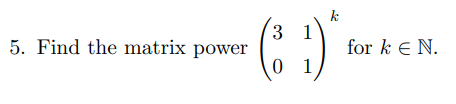k 5. Find the matrix power (3 :) for k € N.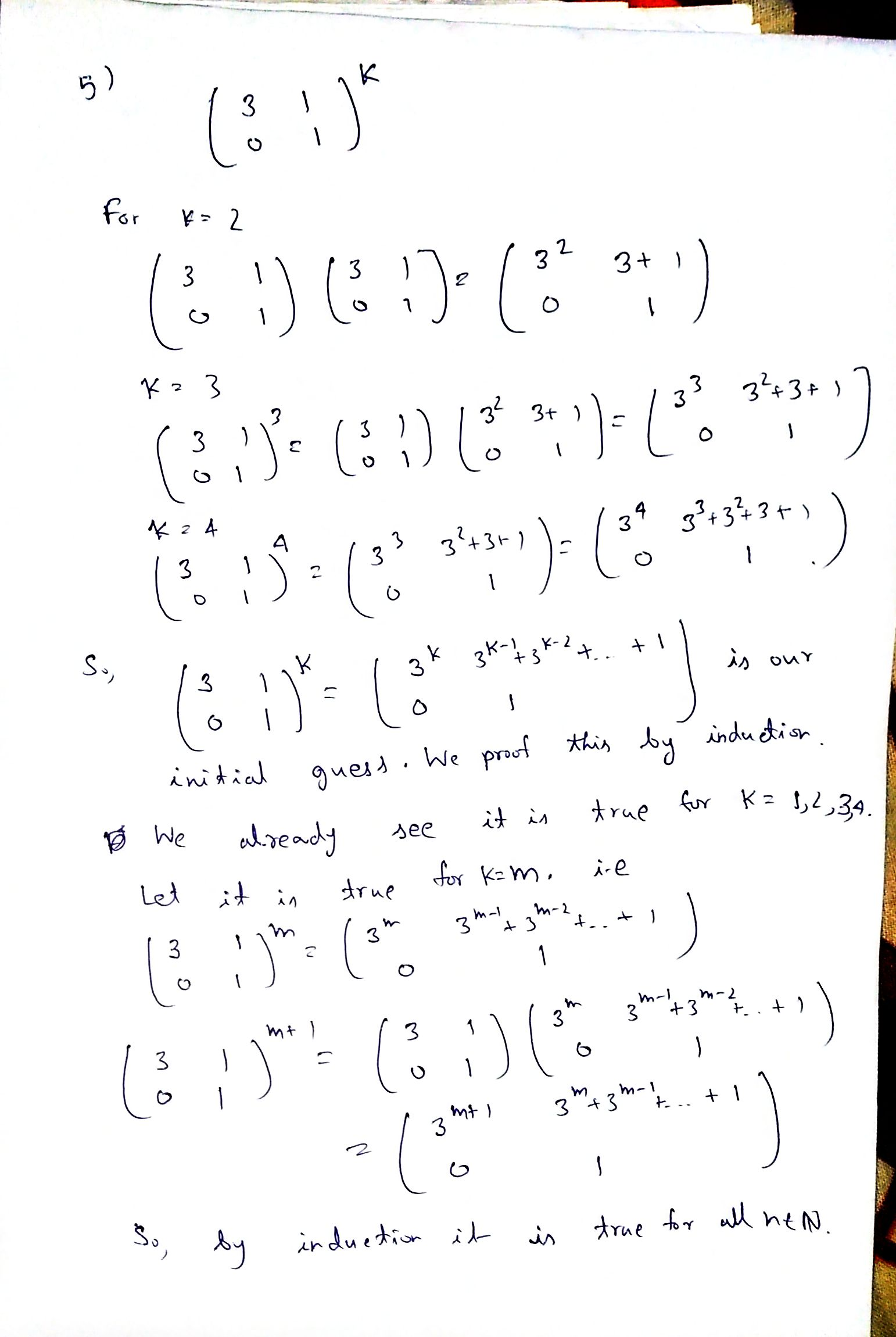#### Earn Coin

Coins can be redeemed for fabulous gifts.

Similar Homework Help Questions
• ### 3.43 Find the formula for the n-th power of this matrix.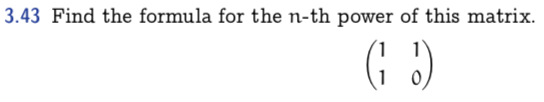3.43 Find the formula for the n-th power of this matrix.

• ### Let A be a diagonalizable n × n matrix and let P be an invertible n...

Let A be a diagonalizable n × n matrix and let P be an invertible n × n matrix such that B = P−1AP is the diagonal form of A. Prove that Ak = PBkP−1, where k is a positive integer. Use the result above to find the indicated power of A. A = −4 0 4 −3 −1 4 −6 0 6 , A5

• ### Let A be a diagonalizable n x n matrix and let P be an invertible n...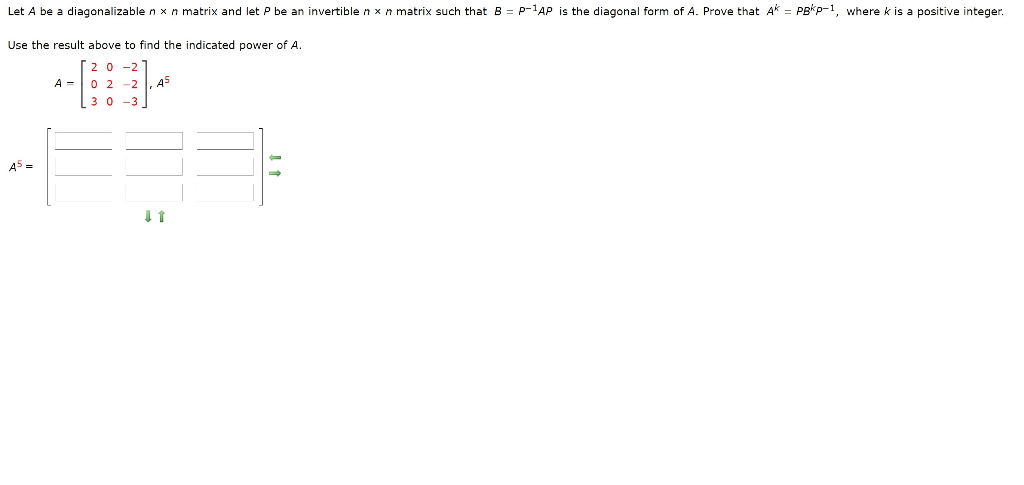Let A be a diagonalizable n x n matrix and let P be an invertible n x n matrix such that B = P-1AP is the diagonal form of A. Prove that Ak = Pekp-1, where k is a positive integer. Use the result above to find the indicated power of A. 0-2 02-2 3 0 -3 ,45 A5 = 11

• ### A is Complex matrix, sps, A is nor（）mal, and A to the power of k =...

A is Complex matrix, sps, A is nor（）mal, and A to the power of k = 0 and k is greater than one Show A= 0;

• ### Let A be a diagonalizable n x n matrix and let P be an invertible n...Let A be a diagonalizable n x n matrix and let P be an invertible n x n matrix such that B = p-1AP is the diagonal form of A. Prove that A* = Pokp-1, where k is a positive integer. Use the result above to find the indicated power of A. 10 18 A = -6 -11 18].46 A = 11

• ###  2. Find the radius of convergence of the power series z" 5(n/2) n=1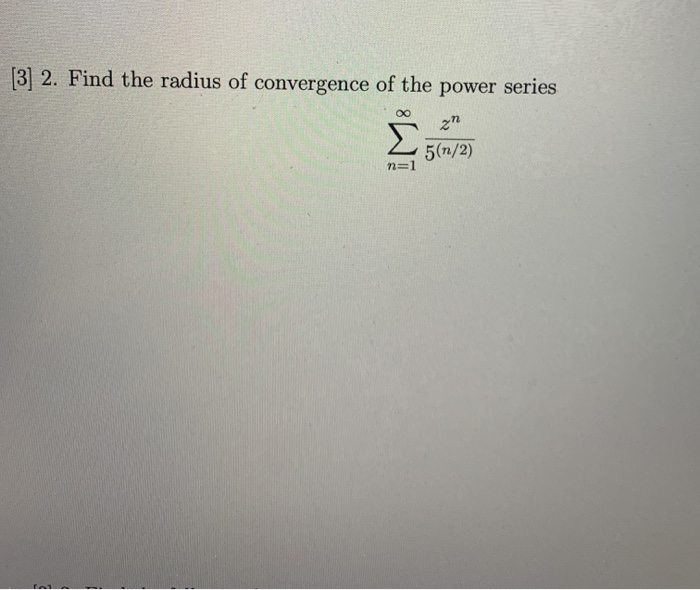2. Find the radius of convergence of the power series z" 5(n/2) n=1

• ### Let A be a diagonalizable n x n matrix and let P be an invertible n...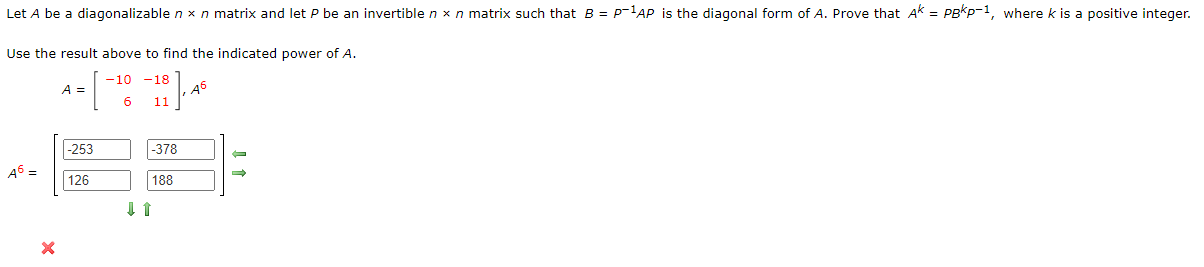Let A be a diagonalizable n x n matrix and let P be an invertible n x n matrix such that B = p-1AP is the diagonal form of A. Prove that Ak = pokp-1, where k is a positive integer. Use the result above to find the indicated power of A. -10 -18 A = 6 11 18].45 -253 -378 A6 = 126 188 11

• ### Q  2. Find the radius of convergence of the power series zn 5(n/2) n=1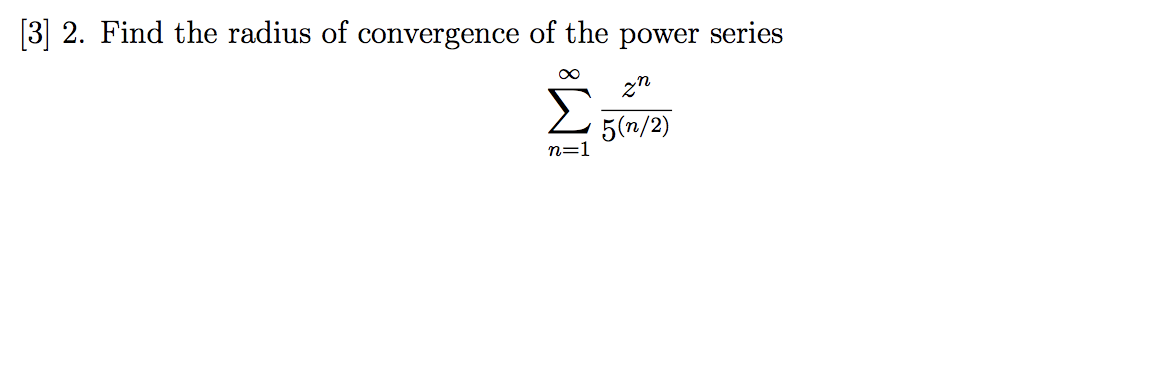Q  2. Find the radius of convergence of the power series zn 5(n/2) n=1

• ### Find the radius of convergence of the following power series: ю 3 zn 5(n/2) n=1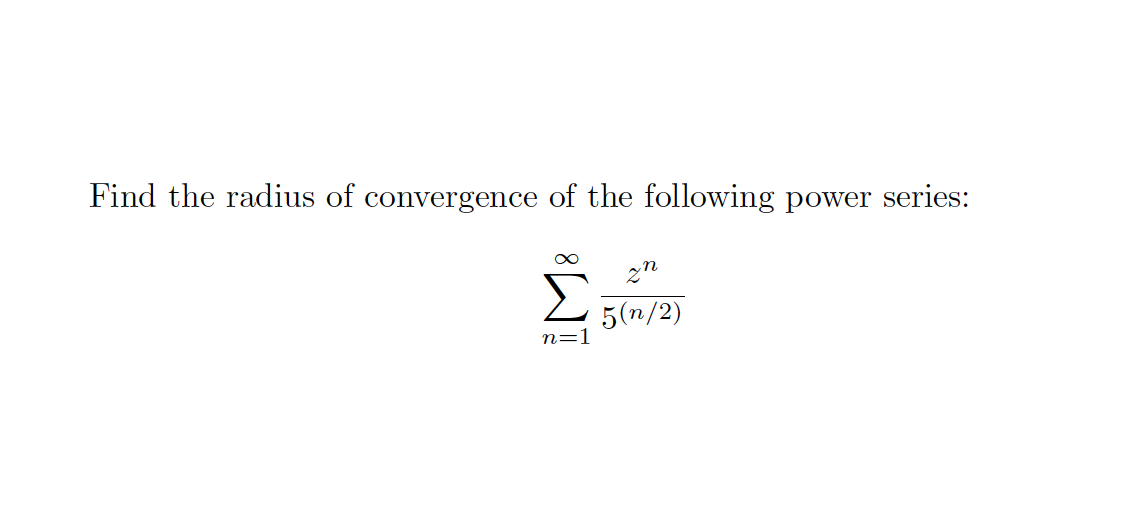Find the radius of convergence of the following power series: ю 3 zn 5(n/2) n=1

• ### Write an algorithm that estimates the desired eigenvalues of an input matrix by using the power...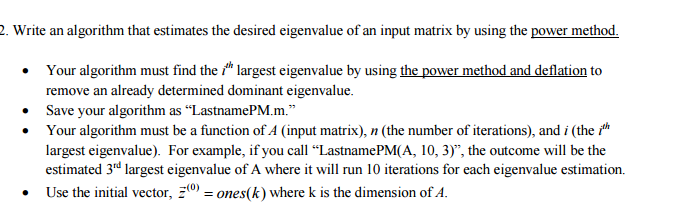Write an algorithm that estimates the desired eigenvalues of an input matrix by using the power method. Your algorithm must find the i^th largest eigenvalues by using the power method and deflation to remove an already determined dominant eigenvalues. Save your algorithm as "LastnamePM.m." Your algorithm must be a function of A (input matrix), n (the number of iterations), and i (the i^th largest eigenvalues). For example, if you call "LastnamePM(A, 10,3)", the outcome will be the estimated 3,d largest...

Free Homework App